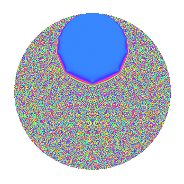# Properties

 Label 3.40.a.aLevel 3 Weight 40 Character orbit 3.a Self dual Yes Analytic conductor 28.902 Analytic rank 1 Dimension 3 CM No Inner twists 1

# Related objects

## Newspace parameters

 Level: $$N$$ = $$3$$ Weight: $$k$$ = $$40$$ Character orbit: $$[\chi]$$ = 3.a (trivial)

## Newform invariants

 Self dual: Yes Analytic conductor: $$28.9018654169$$ Analytic rank: $$1$$ Dimension: $$3$$ Coefficient field: $$\mathbb{Q}[x]/(x^{3} - \cdots)$$ Coefficient ring: $$\Z[a_1, \ldots, a_{5}]$$ Coefficient ring index: $$2^{10}\cdot 3^{6}\cdot 5$$ Fricke sign: $$-1$$ Sato-Tate group: $\mathrm{SU}(2)$

## $q$-expansion

Coefficients of the $$q$$-expansion are expressed in terms of a basis $$1,\beta_1,\beta_2$$ for the coefficient ring described below. We also show the integral $$q$$-expansion of the trace form.

 $$f(q)$$ $$=$$ $$q$$ $$+ ( -369000 - \beta_{1} ) q^{2}$$ $$+ 1162261467 q^{3}$$ $$+ ( 335300075200 + 785144 \beta_{1} + 16 \beta_{2} ) q^{4}$$ $$+ ( 3119016476430 + 32303812 \beta_{1} - 513 \beta_{2} ) q^{5}$$ $$+ ( -428874481323000 - 1162261467 \beta_{1} ) q^{6}$$ $$+ ( 4613961459153368 - 30823389604 \beta_{1} + 348985 \beta_{2} ) q^{7}$$ $$+ ( -508852326783711744 - 484502113856 \beta_{1} - 17712000 \beta_{2} ) q^{8}$$ $$+ 1350851717672992089 q^{9}$$ $$+O(q^{10})$$ $$q$$ $$+(-369000 - \beta_{1}) q^{2}$$ $$+1162261467 q^{3}$$ $$+(335300075200 + 785144 \beta_{1} + 16 \beta_{2}) q^{4}$$ $$+(3119016476430 + 32303812 \beta_{1} - 513 \beta_{2}) q^{5}$$ $$+(-428874481323000 - 1162261467 \beta_{1}) q^{6}$$ $$+(4613961459153368 - 30823389604 \beta_{1} + 348985 \beta_{2}) q^{7}$$ $$+(-508852326783711744 - 484502113856 \beta_{1} - 17712000 \beta_{2}) q^{8}$$ $$+1350851717672992089 q^{9}$$ $$+(-25343199726347478960 - 4627593552014 \beta_{1} - 351748864 \beta_{2}) q^{10}$$ $$+(-13350109203715747932 + 87947509241336 \beta_{1} + 4631921442 \beta_{2}) q^{11}$$ $$+($$$$38\!\cdots\!00$$$$+ 912542617246248 \beta_{1} + 18596183472 \beta_{2}) q^{12}$$ $$+(-$$$$46\!\cdots\!98$$$$- 569679887088520 \beta_{1} - 206263539326 \beta_{2}) q^{13}$$ $$+($$$$21\!\cdots\!32$$$$+ 94200779733928 \beta_{1} + 380851317504 \beta_{2}) q^{14}$$ $$+($$$$36\!\cdots\!10$$$$+ 37545475924812204 \beta_{1} - 596240132571 \beta_{2}) q^{15}$$ $$+($$$$36\!\cdots\!28$$$$+ 690890446516379136 \beta_{1} + 4656654271488 \beta_{2}) q^{16}$$ $$+(-$$$$56\!\cdots\!06$$$$+ 926992962792897096 \beta_{1} - 6009628140594 \beta_{2}) q^{17}$$ $$+(-$$$$49\!\cdots\!00$$$$- 1350851717672992089 \beta_{1}) q^{18}$$ $$+(-$$$$23\!\cdots\!96$$$$+ 2957557631558772120 \beta_{1} - 160826348093766 \beta_{2}) q^{19}$$ $$+($$$$11\!\cdots\!80$$$$+ 17692841649648817552 \beta_{1} + 469278711728352 \beta_{2}) q^{20}$$ $$+($$$$53\!\cdots\!56$$$$- 35824838019057589068 \beta_{1} + 405611818060995 \beta_{2}) q^{21}$$ $$+(-$$$$60\!\cdots\!32$$$$-$$$$13\!\cdots\!48$$$$\beta_{1} - 2897971855497728 \beta_{2}) q^{22}$$ $$+(-$$$$85\!\cdots\!24$$$$-$$$$24\!\cdots\!96$$$$\beta_{1} + 7342398742559922 \beta_{2}) q^{23}$$ $$+(-$$$$59\!\cdots\!48$$$$-$$$$56\!\cdots\!52$$$$\beta_{1} - 20585975103504000 \beta_{2}) q^{24}$$ $$+(-$$$$74\!\cdots\!25$$$$-$$$$53\!\cdots\!20$$$$\beta_{1} + 8936618308826980 \beta_{2}) q^{25}$$ $$+($$$$21\!\cdots\!52$$$$+$$$$96\!\cdots\!66$$$$\beta_{1} + 75502035906725376 \beta_{2}) q^{26}$$ $$+$$$$15\!\cdots\!63$$$$q^{27}$$ $$+(-$$$$10\!\cdots\!16$$$$-$$$$13\!\cdots\!64$$$$\beta_{1} - 315943026832013952 \beta_{2}) q^{28}$$ $$+(-$$$$15\!\cdots\!62$$$$+$$$$18\!\cdots\!44$$$$\beta_{1} + 119886169875921837 \beta_{2}) q^{29}$$ $$+(-$$$$29\!\cdots\!20$$$$-$$$$53\!\cdots\!38$$$$\beta_{1} - 408824150688223488 \beta_{2}) q^{30}$$ $$+(-$$$$94\!\cdots\!96$$$$+$$$$90\!\cdots\!60$$$$\beta_{1} + 2713599485454965797 \beta_{2}) q^{31}$$ $$+(-$$$$37\!\cdots\!92$$$$-$$$$49\!\cdots\!28$$$$\beta_{1} - 2815744285881851904 \beta_{2}) q^{32}$$ $$+(-$$$$15\!\cdots\!44$$$$+$$$$10\!\cdots\!12$$$$\beta_{1} + 5383503810207675414 \beta_{2}) q^{33}$$ $$+(-$$$$67\!\cdots\!00$$$$-$$$$18\!\cdots\!46$$$$\beta_{1} - 12897652529867331072 \beta_{2}) q^{34}$$ $$+(-$$$$92\!\cdots\!00$$$$+$$$$64\!\cdots\!20$$$$\beta_{1} - 14321872082373654330 \beta_{2}) q^{35}$$ $$+($$$$45\!\cdots\!00$$$$+$$$$10\!\cdots\!16$$$$\beta_{1} + 21613627482767873424 \beta_{2}) q^{36}$$ $$+(-$$$$17\!\cdots\!62$$$$+$$$$19\!\cdots\!00$$$$\beta_{1} + 85573013785692403224 \beta_{2}) q^{37}$$ $$+(-$$$$13\!\cdots\!88$$$$+$$$$48\!\cdots\!24$$$$\beta_{1} + 4442002987126795776 \beta_{2}) q^{38}$$ $$+(-$$$$53\!\cdots\!66$$$$-$$$$66\!\cdots\!40$$$$\beta_{1} -$$$$23\!\cdots\!42$$$$\beta_{2}) q^{39}$$ $$+(-$$$$34\!\cdots\!80$$$$-$$$$26\!\cdots\!12$$$$\beta_{1} -$$$$24\!\cdots\!12$$$$\beta_{2}) q^{40}$$ $$+($$$$15\!\cdots\!94$$$$-$$$$38\!\cdots\!24$$$$\beta_{1} +$$$$53\!\cdots\!38$$$$\beta_{2}) q^{41}$$ $$+($$$$24\!\cdots\!44$$$$+$$$$10\!\cdots\!76$$$$\beta_{1} +$$$$44\!\cdots\!68$$$$\beta_{2}) q^{42}$$ $$+(-$$$$49\!\cdots\!96$$$$-$$$$68\!\cdots\!24$$$$\beta_{1} -$$$$61\!\cdots\!18$$$$\beta_{2}) q^{43}$$ $$+($$$$12\!\cdots\!16$$$$+$$$$13\!\cdots\!40$$$$\beta_{1} +$$$$48\!\cdots\!40$$$$\beta_{2}) q^{44}$$ $$+($$$$42\!\cdots\!70$$$$+$$$$43\!\cdots\!68$$$$\beta_{1} -$$$$69\!\cdots\!57$$$$\beta_{2}) q^{45}$$ $$+($$$$21\!\cdots\!24$$$$+$$$$17\!\cdots\!12$$$$\beta_{1} +$$$$15\!\cdots\!04$$$$\beta_{2}) q^{46}$$ $$+(-$$$$43\!\cdots\!48$$$$-$$$$20\!\cdots\!40$$$$\beta_{1} -$$$$99\!\cdots\!42$$$$\beta_{2}) q^{47}$$ $$+($$$$42\!\cdots\!76$$$$+$$$$80\!\cdots\!12$$$$\beta_{1} +$$$$54\!\cdots\!96$$$$\beta_{2}) q^{48}$$ $$+(-$$$$44\!\cdots\!71$$$$-$$$$74\!\cdots\!80$$$$\beta_{1} +$$$$16\!\cdots\!36$$$$\beta_{2}) q^{49}$$ $$+($$$$67\!\cdots\!00$$$$+$$$$75\!\cdots\!65$$$$\beta_{1} +$$$$57\!\cdots\!40$$$$\beta_{2}) q^{50}$$ $$+(-$$$$65\!\cdots\!02$$$$+$$$$10\!\cdots\!32$$$$\beta_{1} -$$$$69\!\cdots\!98$$$$\beta_{2}) q^{51}$$ $$+(-$$$$54\!\cdots\!76$$$$-$$$$76\!\cdots\!84$$$$\beta_{1} -$$$$65\!\cdots\!24$$$$\beta_{2}) q^{52}$$ $$+(-$$$$36\!\cdots\!14$$$$+$$$$10\!\cdots\!24$$$$\beta_{1} +$$$$18\!\cdots\!37$$$$\beta_{2}) q^{53}$$ $$+(-$$$$57\!\cdots\!00$$$$-$$$$15\!\cdots\!63$$$$\beta_{1}) q^{54}$$ $$+(-$$$$50\!\cdots\!20$$$$+$$$$25\!\cdots\!52$$$$\beta_{1} +$$$$11\!\cdots\!52$$$$\beta_{2}) q^{55}$$ $$+($$$$21\!\cdots\!00$$$$+$$$$23\!\cdots\!44$$$$\beta_{1} +$$$$10\!\cdots\!84$$$$\beta_{2}) q^{56}$$ $$+(-$$$$26\!\cdots\!32$$$$+$$$$34\!\cdots\!40$$$$\beta_{1} -$$$$18\!\cdots\!22$$$$\beta_{2}) q^{57}$$ $$+(-$$$$79\!\cdots\!76$$$$+$$$$50\!\cdots\!70$$$$\beta_{1} -$$$$32\!\cdots\!76$$$$\beta_{2}) q^{58}$$ $$+($$$$11\!\cdots\!16$$$$-$$$$40\!\cdots\!52$$$$\beta_{1} +$$$$26\!\cdots\!44$$$$\beta_{2}) q^{59}$$ $$+($$$$12\!\cdots\!60$$$$+$$$$20\!\cdots\!84$$$$\beta_{1} +$$$$54\!\cdots\!84$$$$\beta_{2}) q^{60}$$ $$+(-$$$$87\!\cdots\!34$$$$-$$$$59\!\cdots\!00$$$$\beta_{1} -$$$$18\!\cdots\!56$$$$\beta_{2}) q^{61}$$ $$+(-$$$$32\!\cdots\!04$$$$-$$$$64\!\cdots\!80$$$$\beta_{1} -$$$$23\!\cdots\!92$$$$\beta_{2}) q^{62}$$ $$+($$$$62\!\cdots\!52$$$$-$$$$41\!\cdots\!56$$$$\beta_{1} +$$$$47\!\cdots\!65$$$$\beta_{2}) q^{63}$$ $$+($$$$30\!\cdots\!68$$$$+$$$$26\!\cdots\!08$$$$\beta_{1} +$$$$62\!\cdots\!28$$$$\beta_{2}) q^{64}$$ $$+($$$$86\!\cdots\!20$$$$-$$$$30\!\cdots\!52$$$$\beta_{1} -$$$$71\!\cdots\!02$$$$\beta_{2}) q^{65}$$ $$+(-$$$$70\!\cdots\!44$$$$-$$$$15\!\cdots\!16$$$$\beta_{1} -$$$$33\!\cdots\!76$$$$\beta_{2}) q^{66}$$ $$+(-$$$$66\!\cdots\!96$$$$+$$$$26\!\cdots\!20$$$$\beta_{1} -$$$$14\!\cdots\!60$$$$\beta_{2}) q^{67}$$ $$+($$$$42\!\cdots\!00$$$$+$$$$54\!\cdots\!12$$$$\beta_{1} +$$$$10\!\cdots\!40$$$$\beta_{2}) q^{68}$$ $$+(-$$$$99\!\cdots\!08$$$$-$$$$28\!\cdots\!32$$$$\beta_{1} +$$$$85\!\cdots\!74$$$$\beta_{2}) q^{69}$$ $$+(-$$$$14\!\cdots\!00$$$$+$$$$98\!\cdots\!60$$$$\beta_{1} -$$$$57\!\cdots\!40$$$$\beta_{2}) q^{70}$$ $$+(-$$$$10\!\cdots\!48$$$$-$$$$25\!\cdots\!16$$$$\beta_{1} +$$$$16\!\cdots\!74$$$$\beta_{2}) q^{71}$$ $$+(-$$$$68\!\cdots\!16$$$$-$$$$65\!\cdots\!84$$$$\beta_{1} -$$$$23\!\cdots\!00$$$$\beta_{2}) q^{72}$$ $$+(-$$$$12\!\cdots\!74$$$$-$$$$16\!\cdots\!88$$$$\beta_{1} +$$$$37\!\cdots\!20$$$$\beta_{2}) q^{73}$$ $$+(-$$$$84\!\cdots\!08$$$$-$$$$10\!\cdots\!50$$$$\beta_{1} -$$$$59\!\cdots\!44$$$$\beta_{2}) q^{74}$$ $$+(-$$$$86\!\cdots\!75$$$$-$$$$62\!\cdots\!40$$$$\beta_{1} +$$$$10\!\cdots\!60$$$$\beta_{2}) q^{75}$$ $$+(-$$$$18\!\cdots\!56$$$$-$$$$23\!\cdots\!16$$$$\beta_{1} +$$$$97\!\cdots\!68$$$$\beta_{2}) q^{76}$$ $$+(-$$$$33\!\cdots\!24$$$$-$$$$20\!\cdots\!64$$$$\beta_{1} -$$$$58\!\cdots\!76$$$$\beta_{2}) q^{77}$$ $$+($$$$24\!\cdots\!84$$$$+$$$$11\!\cdots\!22$$$$\beta_{1} +$$$$87\!\cdots\!92$$$$\beta_{2}) q^{78}$$ $$+(-$$$$34\!\cdots\!00$$$$+$$$$73\!\cdots\!44$$$$\beta_{1} -$$$$36\!\cdots\!91$$$$\beta_{2}) q^{79}$$ $$+($$$$15\!\cdots\!20$$$$+$$$$10\!\cdots\!88$$$$\beta_{1} +$$$$24\!\cdots\!88$$$$\beta_{2}) q^{80}$$ $$+$$$$18\!\cdots\!21$$$$q^{81}$$ $$+(-$$$$55\!\cdots\!84$$$$-$$$$28\!\cdots\!82$$$$\beta_{1} -$$$$16\!\cdots\!44$$$$\beta_{2}) q^{82}$$ $$+($$$$23\!\cdots\!80$$$$+$$$$12\!\cdots\!08$$$$\beta_{1} -$$$$53\!\cdots\!30$$$$\beta_{2}) q^{83}$$ $$+(-$$$$12\!\cdots\!72$$$$-$$$$15\!\cdots\!88$$$$\beta_{1} -$$$$36\!\cdots\!84$$$$\beta_{2}) q^{84}$$ $$+($$$$25\!\cdots\!20$$$$-$$$$13\!\cdots\!52$$$$\beta_{1} +$$$$44\!\cdots\!98$$$$\beta_{2}) q^{85}$$ $$+($$$$53\!\cdots\!68$$$$+$$$$47\!\cdots\!36$$$$\beta_{1} +$$$$12\!\cdots\!92$$$$\beta_{2}) q^{86}$$ $$+(-$$$$17\!\cdots\!54$$$$+$$$$21\!\cdots\!48$$$$\beta_{1} +$$$$13\!\cdots\!79$$$$\beta_{2}) q^{87}$$ $$+(-$$$$11\!\cdots\!84$$$$-$$$$12\!\cdots\!72$$$$\beta_{1} -$$$$71\!\cdots\!16$$$$\beta_{2}) q^{88}$$ $$+($$$$45\!\cdots\!14$$$$-$$$$22\!\cdots\!72$$$$\beta_{1} +$$$$34\!\cdots\!12$$$$\beta_{2}) q^{89}$$ $$+(-$$$$34\!\cdots\!40$$$$-$$$$62\!\cdots\!46$$$$\beta_{1} -$$$$47\!\cdots\!96$$$$\beta_{2}) q^{90}$$ $$+(-$$$$86\!\cdots\!36$$$$+$$$$28\!\cdots\!04$$$$\beta_{1} -$$$$90\!\cdots\!14$$$$\beta_{2}) q^{91}$$ $$+(-$$$$45\!\cdots\!12$$$$-$$$$12\!\cdots\!56$$$$\beta_{1} -$$$$48\!\cdots\!52$$$$\beta_{2}) q^{92}$$ $$+(-$$$$10\!\cdots\!32$$$$+$$$$10\!\cdots\!20$$$$\beta_{1} +$$$$31\!\cdots\!99$$$$\beta_{2}) q^{93}$$ $$+($$$$15\!\cdots\!84$$$$+$$$$31\!\cdots\!04$$$$\beta_{1} +$$$$64\!\cdots\!92$$$$\beta_{2}) q^{94}$$ $$+($$$$15\!\cdots\!80$$$$-$$$$22\!\cdots\!08$$$$\beta_{1} +$$$$61\!\cdots\!92$$$$\beta_{2}) q^{95}$$ $$+(-$$$$43\!\cdots\!64$$$$-$$$$57\!\cdots\!76$$$$\beta_{1} -$$$$32\!\cdots\!68$$$$\beta_{2}) q^{96}$$ $$+(-$$$$44\!\cdots\!06$$$$+$$$$35\!\cdots\!36$$$$\beta_{1} -$$$$27\!\cdots\!24$$$$\beta_{2}) q^{97}$$ $$+($$$$57\!\cdots\!28$$$$-$$$$30\!\cdots\!77$$$$\beta_{1} +$$$$66\!\cdots\!64$$$$\beta_{2}) q^{98}$$ $$+(-$$$$18\!\cdots\!48$$$$+$$$$11\!\cdots\!04$$$$\beta_{1} +$$$$62\!\cdots\!38$$$$\beta_{2}) q^{99}$$ $$+O(q^{100})$$ $$\operatorname{Tr}(f)(q)$$ $$=$$ $$3q$$ $$\mathstrut -\mathstrut 1107000q^{2}$$ $$\mathstrut +\mathstrut 3486784401q^{3}$$ $$\mathstrut +\mathstrut 1005900225600q^{4}$$ $$\mathstrut +\mathstrut 9357049429290q^{5}$$ $$\mathstrut -\mathstrut 1286623443969000q^{6}$$ $$\mathstrut +\mathstrut 13841884377460104q^{7}$$ $$\mathstrut -\mathstrut 1526556980351135232q^{8}$$ $$\mathstrut +\mathstrut 4052555153018976267q^{9}$$ $$\mathstrut +\mathstrut O(q^{10})$$ $$3q$$ $$\mathstrut -\mathstrut 1107000q^{2}$$ $$\mathstrut +\mathstrut 3486784401q^{3}$$ $$\mathstrut +\mathstrut 1005900225600q^{4}$$ $$\mathstrut +\mathstrut 9357049429290q^{5}$$ $$\mathstrut -\mathstrut 1286623443969000q^{6}$$ $$\mathstrut +\mathstrut 13841884377460104q^{7}$$ $$\mathstrut -\mathstrut 1526556980351135232q^{8}$$ $$\mathstrut +\mathstrut 4052555153018976267q^{9}$$ $$\mathstrut -\mathstrut 76029599179042436880q^{10}$$ $$\mathstrut -\mathstrut 40050327611147243796q^{11}$$ $$\mathstrut +\mathstrut$$$$11\!\cdots\!00$$$$q^{12}$$ $$\mathstrut -\mathstrut$$$$13\!\cdots\!94$$$$q^{13}$$ $$\mathstrut +\mathstrut$$$$64\!\cdots\!96$$$$q^{14}$$ $$\mathstrut +\mathstrut$$$$10\!\cdots\!30$$$$q^{15}$$ $$\mathstrut +\mathstrut$$$$10\!\cdots\!84$$$$q^{16}$$ $$\mathstrut -\mathstrut$$$$16\!\cdots\!18$$$$q^{17}$$ $$\mathstrut -\mathstrut$$$$14\!\cdots\!00$$$$q^{18}$$ $$\mathstrut -\mathstrut$$$$69\!\cdots\!88$$$$q^{19}$$ $$\mathstrut +\mathstrut$$$$33\!\cdots\!40$$$$q^{20}$$ $$\mathstrut +\mathstrut$$$$16\!\cdots\!68$$$$q^{21}$$ $$\mathstrut -\mathstrut$$$$18\!\cdots\!96$$$$q^{22}$$ $$\mathstrut -\mathstrut$$$$25\!\cdots\!72$$$$q^{23}$$ $$\mathstrut -\mathstrut$$$$17\!\cdots\!44$$$$q^{24}$$ $$\mathstrut -\mathstrut$$$$22\!\cdots\!75$$$$q^{25}$$ $$\mathstrut +\mathstrut$$$$64\!\cdots\!56$$$$q^{26}$$ $$\mathstrut +\mathstrut$$$$47\!\cdots\!89$$$$q^{27}$$ $$\mathstrut -\mathstrut$$$$31\!\cdots\!48$$$$q^{28}$$ $$\mathstrut -\mathstrut$$$$46\!\cdots\!86$$$$q^{29}$$ $$\mathstrut -\mathstrut$$$$88\!\cdots\!60$$$$q^{30}$$ $$\mathstrut -\mathstrut$$$$28\!\cdots\!88$$$$q^{31}$$ $$\mathstrut -\mathstrut$$$$11\!\cdots\!76$$$$q^{32}$$ $$\mathstrut -\mathstrut$$$$46\!\cdots\!32$$$$q^{33}$$ $$\mathstrut -\mathstrut$$$$20\!\cdots\!00$$$$q^{34}$$ $$\mathstrut -\mathstrut$$$$27\!\cdots\!00$$$$q^{35}$$ $$\mathstrut +\mathstrut$$$$13\!\cdots\!00$$$$q^{36}$$ $$\mathstrut -\mathstrut$$$$52\!\cdots\!86$$$$q^{37}$$ $$\mathstrut -\mathstrut$$$$40\!\cdots\!64$$$$q^{38}$$ $$\mathstrut -\mathstrut$$$$16\!\cdots\!98$$$$q^{39}$$ $$\mathstrut -\mathstrut$$$$10\!\cdots\!40$$$$q^{40}$$ $$\mathstrut +\mathstrut$$$$47\!\cdots\!82$$$$q^{41}$$ $$\mathstrut +\mathstrut$$$$74\!\cdots\!32$$$$q^{42}$$ $$\mathstrut -\mathstrut$$$$14\!\cdots\!88$$$$q^{43}$$ $$\mathstrut +\mathstrut$$$$38\!\cdots\!48$$$$q^{44}$$ $$\mathstrut +\mathstrut$$$$12\!\cdots\!10$$$$q^{45}$$ $$\mathstrut +\mathstrut$$$$64\!\cdots\!72$$$$q^{46}$$ $$\mathstrut -\mathstrut$$$$13\!\cdots\!44$$$$q^{47}$$ $$\mathstrut +\mathstrut$$$$12\!\cdots\!28$$$$q^{48}$$ $$\mathstrut -\mathstrut$$$$13\!\cdots\!13$$$$q^{49}$$ $$\mathstrut +\mathstrut$$$$20\!\cdots\!00$$$$q^{50}$$ $$\mathstrut -\mathstrut$$$$19\!\cdots\!06$$$$q^{51}$$ $$\mathstrut -\mathstrut$$$$16\!\cdots\!28$$$$q^{52}$$ $$\mathstrut -\mathstrut$$$$11\!\cdots\!42$$$$q^{53}$$ $$\mathstrut -\mathstrut$$$$17\!\cdots\!00$$$$q^{54}$$ $$\mathstrut -\mathstrut$$$$15\!\cdots\!60$$$$q^{55}$$ $$\mathstrut +\mathstrut$$$$63\!\cdots\!00$$$$q^{56}$$ $$\mathstrut -\mathstrut$$$$80\!\cdots\!96$$$$q^{57}$$ $$\mathstrut -\mathstrut$$$$23\!\cdots\!28$$$$q^{58}$$ $$\mathstrut +\mathstrut$$$$35\!\cdots\!48$$$$q^{59}$$ $$\mathstrut +\mathstrut$$$$38\!\cdots\!80$$$$q^{60}$$ $$\mathstrut -\mathstrut$$$$26\!\cdots\!02$$$$q^{61}$$ $$\mathstrut -\mathstrut$$$$98\!\cdots\!12$$$$q^{62}$$ $$\mathstrut +\mathstrut$$$$18\!\cdots\!56$$$$q^{63}$$ $$\mathstrut +\mathstrut$$$$92\!\cdots\!04$$$$q^{64}$$ $$\mathstrut +\mathstrut$$$$26\!\cdots\!60$$$$q^{65}$$ $$\mathstrut -\mathstrut$$$$21\!\cdots\!32$$$$q^{66}$$ $$\mathstrut -\mathstrut$$$$20\!\cdots\!88$$$$q^{67}$$ $$\mathstrut +\mathstrut$$$$12\!\cdots\!00$$$$q^{68}$$ $$\mathstrut -\mathstrut$$$$29\!\cdots\!24$$$$q^{69}$$ $$\mathstrut -\mathstrut$$$$43\!\cdots\!00$$$$q^{70}$$ $$\mathstrut -\mathstrut$$$$31\!\cdots\!44$$$$q^{71}$$ $$\mathstrut -\mathstrut$$$$20\!\cdots\!48$$$$q^{72}$$ $$\mathstrut -\mathstrut$$$$38\!\cdots\!22$$$$q^{73}$$ $$\mathstrut -\mathstrut$$$$25\!\cdots\!24$$$$q^{74}$$ $$\mathstrut -\mathstrut$$$$25\!\cdots\!25$$$$q^{75}$$ $$\mathstrut -\mathstrut$$$$55\!\cdots\!68$$$$q^{76}$$ $$\mathstrut -\mathstrut$$$$99\!\cdots\!72$$$$q^{77}$$ $$\mathstrut +\mathstrut$$$$74\!\cdots\!52$$$$q^{78}$$ $$\mathstrut -\mathstrut$$$$10\!\cdots\!00$$$$q^{79}$$ $$\mathstrut +\mathstrut$$$$45\!\cdots\!60$$$$q^{80}$$ $$\mathstrut +\mathstrut$$$$54\!\cdots\!63$$$$q^{81}$$ $$\mathstrut -\mathstrut$$$$16\!\cdots\!52$$$$q^{82}$$ $$\mathstrut +\mathstrut$$$$69\!\cdots\!40$$$$q^{83}$$ $$\mathstrut -\mathstrut$$$$36\!\cdots\!16$$$$q^{84}$$ $$\mathstrut +\mathstrut$$$$76\!\cdots\!60$$$$q^{85}$$ $$\mathstrut +\mathstrut$$$$15\!\cdots\!04$$$$q^{86}$$ $$\mathstrut -\mathstrut$$$$53\!\cdots\!62$$$$q^{87}$$ $$\mathstrut -\mathstrut$$$$34\!\cdots\!52$$$$q^{88}$$ $$\mathstrut +\mathstrut$$$$13\!\cdots\!42$$$$q^{89}$$ $$\mathstrut -\mathstrut$$$$10\!\cdots\!20$$$$q^{90}$$ $$\mathstrut -\mathstrut$$$$25\!\cdots\!08$$$$q^{91}$$ $$\mathstrut -\mathstrut$$$$13\!\cdots\!36$$$$q^{92}$$ $$\mathstrut -\mathstrut$$$$32\!\cdots\!96$$$$q^{93}$$ $$\mathstrut +\mathstrut$$$$45\!\cdots\!52$$$$q^{94}$$ $$\mathstrut +\mathstrut$$$$46\!\cdots\!40$$$$q^{95}$$ $$\mathstrut -\mathstrut$$$$12\!\cdots\!92$$$$q^{96}$$ $$\mathstrut -\mathstrut$$$$13\!\cdots\!18$$$$q^{97}$$ $$\mathstrut +\mathstrut$$$$17\!\cdots\!84$$$$q^{98}$$ $$\mathstrut -\mathstrut$$$$54\!\cdots\!44$$$$q^{99}$$ $$\mathstrut +\mathstrut O(q^{100})$$

Basis of coefficient ring in terms of a root $$\nu$$ of $$x^{3}\mathstrut -\mathstrut$$ $$216694123$$ $$x\mathstrut -\mathstrut$$ $$94580724378$$:

 $$\beta_{0}$$ $$=$$ $$1$$ $$\beta_{1}$$ $$=$$ $$72 \nu$$ $$\beta_{2}$$ $$=$$ $$324 \nu^{2} - 212148 \nu - 46805930568$$
 $$1$$ $$=$$ $$\beta_0$$ $$\nu$$ $$=$$ $$\beta_{1}$$$$/72$$ $$\nu^{2}$$ $$=$$ $$($$$$2$$ $$\beta_{2}\mathstrut +\mathstrut$$ $$5893$$ $$\beta_{1}\mathstrut +\mathstrut$$ $$93611861136$$$$)/648$$

## Embeddings

For each embedding $$\iota_m$$ of the coefficient field, the values $$\iota_m(a_n)$$ are shown below.

For more information on an embedded modular form you can click on its label.

Label $$\iota_m(\nu)$$ $$a_{2}$$ $$a_{3}$$ $$a_{4}$$ $$a_{5}$$ $$a_{6}$$ $$a_{7}$$ $$a_{8}$$ $$a_{9}$$ $$a_{10}$$
1.1
 14934.1 −436.856 −14497.2
−1.44426e6 1.16226e9 1.53612e12 2.64208e13 −1.67860e15 −2.07513e16 −1.42456e18 1.35085e18 −3.81584e19
1.2 −337546. 1.16226e9 −4.35818e11 2.60351e13 −3.92317e14 −1.06972e16 3.32677e17 1.35085e18 −8.78806e18
1.3 674802. 1.16226e9 −9.43987e10 −4.30989e13 7.84296e14 4.52904e16 −4.34676e17 1.35085e18 −2.90832e19
 $$n$$: e.g. 2-40 or 990-1000 Significant digits: Format: Complex embeddings Normalized embeddings Satake parameters Satake angles

## Inner twists

This newform does not admit any (nontrivial) inner twists.

## Atkin-Lehner signs

$$p$$ Sign
$$3$$ $$-1$$

## Hecke kernels

This newform can be constructed as the kernel of the linear operator $$T_{2}^{3}$$ $$\mathstrut +\mathstrut 1107000 T_{2}^{2}$$ $$\mathstrut -\mathstrut 714859333632 T_{2}$$ $$\mathstrut -\mathstrut$$$$32\!\cdots\!56$$ acting on $$S_{40}^{\mathrm{new}}(\Gamma_0(3))$$.